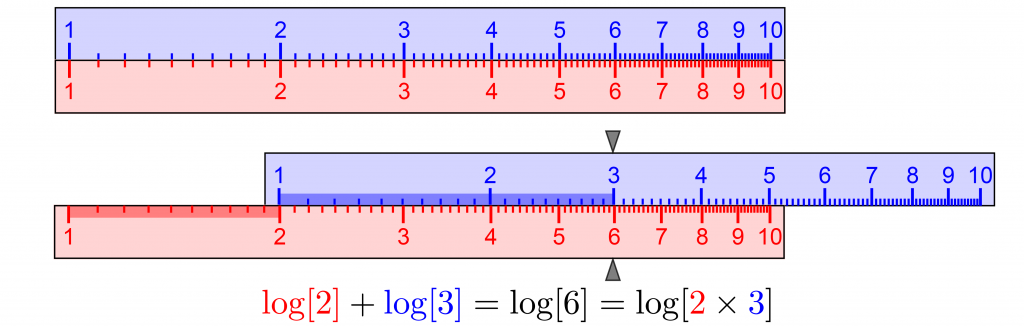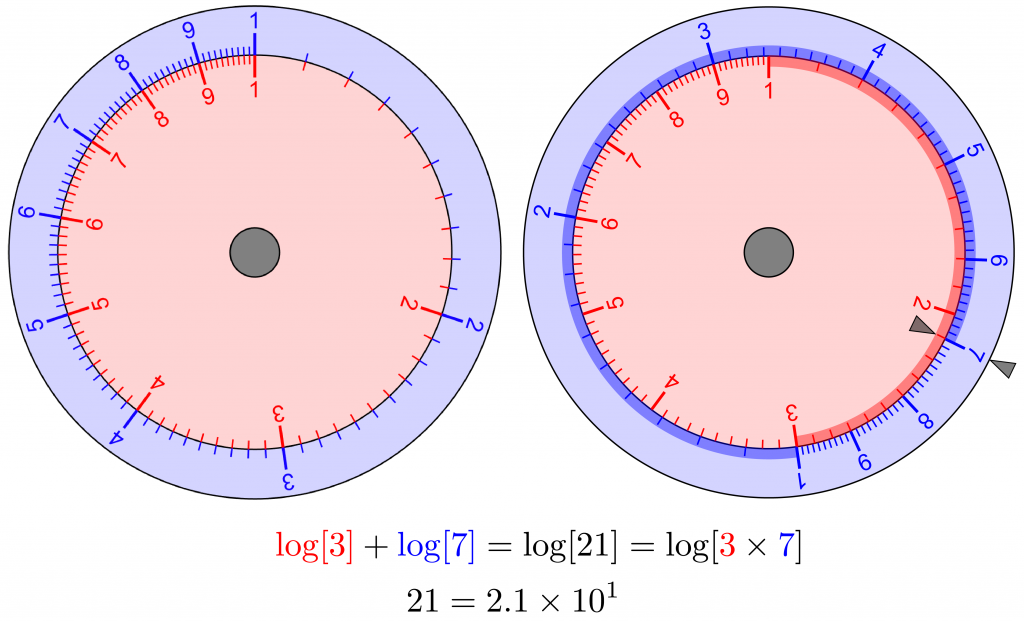# Slide Rule Theory

Slide rules were the analog computers that ruled science and engineering for 400 years. Their brilliant innovation was using logarithms to convert multiplication and division to addition and subtraction,

$$\log xy = \log x + \log y$$

and

$$\log \frac{x}{y} = \log x - \log y.$$

Slide rules feature logarithmic scales that slide past each other. For straight slide rules, logarithms of the numbers are proportional to their distances along them. To multiply $2\times 3 = 6$, as below, slide the upper (blue) scale from 1 to 2 along the lower (red) scale, add the distance from 1 to 3 along the upper (blue) scale, and read the product from the lower (red) scale.Logarithms of the numbers are proportional to their distances along the straight slide rule scales. Slide the upper (blue) scale to multiply numbers by adding these distances.

For circular slide rules, logarithms of the numbers are proportional to their distances around them. To multiply $3\times 7 = 21$, as below, rotate the outer (blue) scale from 1 to 3 around the inner (red)  scale, add the distance from 1 to 7 around the outer (blue) scale, and read the product from the inner (red) scale. Circular slide rules eliminate off-scale calculations because they naturally wrap around, with each wrap multiplying (or dividing) the numbers by 10.Logarithms of the numbers are proportional to their distances around the circular slide rule scales. Rotate the outer (blue) scale to multiply numbers by adding these distances.## About John F. Lindner

John F. Lindner was born in Sleepy Hollow, New York, and educated at the University of Vermont and Caltech. He is an emeritus professor of physics and astronomy at The College of Wooster and a visiting professor at North Carolina State University. He has enjoyed multiple yearlong sabbaticals at Georgia Tech, University of Portland, University of Hawai'i, and NCSU. His research interests include nonlinear dynamics, celestial mechanics, and neural networks.
This entry was posted in Mathematics. Bookmark the permalink.